Most Affordable JEE | NEET | 8,9,10 Preparation by Kota's Top IITian Doctor Faculties

# NCERT Solutions for Class 11 Chemistry chapter 12 Organic Chemistry - Some Basic Principles and Techniques PDF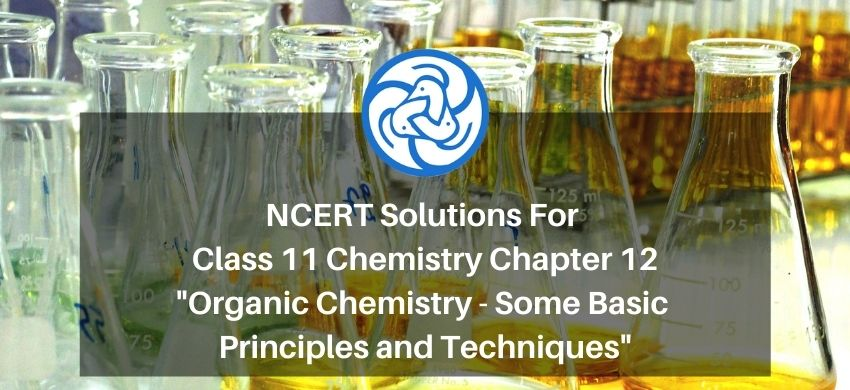# NCERT Solutions for Class 11 Chemistry chapter 12 Organic Chemistry - Some Basic Principles and Techniques PDF

Hey, are you a class 11 student and looking for ways to download NCERT Solutions for Class 11 Chemistry chapter 12 Organic Chemistry - Some Basic Principles and Techniques PDF? If yes. Then read this post till the end.

In this article, we have listed NCERT Solutions for Class 11 Chemistry chapter 12 Organic Chemistry - Some Basic Principles and Techniques in PDF that are prepared by Kota’s top IITian’s Faculties by keeping Simplicity in mind.

If you want to learn and understand class 11 Chemistry chapter 11 "Organic Chemistry - Some Basic Principles and Techniques" in an easy way then you can use these solutions PDF.

NCERT Solutions helps students to Practice important concepts of subjects easily. Class 11 Chemistry solutions provide detailed explanations of all the NCERT questions that students can use to clear their doubts instantly.

If you want to score high in your class 11 Chemistry Exam then it is very important for you to have a good knowledge of all the important topics, so to learn and practice those topics you can use eSaral NCERT Solutions.

In this article, we have listed NCERT Solutions for Class 11 Chemistry chapter 12 Organic Chemistry - Some Basic Principles and Techniques PDF that you can download to start your preparations anytime.

So, without wasting more time Let’s start.

### Download NCERT Solutions for Class 11 Chemistry chapter 12 Organic Chemistry - Some Basic Principles and Techniques PDF

Question 1. What are hybridisation states of each carbon atom in the following compounds?

$\mathrm{CH}_{2}=\mathrm{C}=0, \mathrm{CH}_{3} \mathrm{CH}=\mathrm{CH}_{2}$

$\left(\mathrm{CH}_{3}\right)_{2} \mathrm{CO}, \mathrm{CH}_{2}=\mathrm{CHCN}, \mathrm{C}_{6} \mathrm{H}_{6}$

Solution: Hybridisation $=\sigma$ bond $+$ lone pair

If $2 \sigma=\mathrm{sp}$

$3 \sigma=s p^{2}$

$4 \sigma=\mathrm{sp}^{3}$

(i)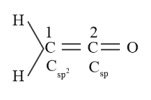C $-1$ is sp $^{2}$ hybridised

C $-2$ is sp hybridized

(ii)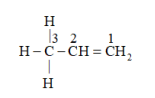$\mathrm{C}-3$ is sp $^{3}$ hybridized

C $-2$ is sp $^{2}$ hybridized

$\mathrm{C}-1$ is sp $^{2}$ hybridized

(iii)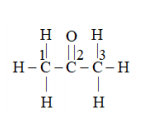$\mathrm{C}-1$ and $\mathrm{C}-3$ are $\mathrm{sp}^{3}$ hybridized

$\mathrm{C}-2$ is $\mathrm{sp}^{2}$ hybridized

(iv)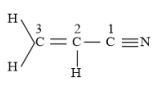$\mathrm{C}-3$ is $\mathrm{sp}^{2}$ hybridized

$\mathrm{C}-2$ is $\mathrm{sp}^{2}$ hybridized

C $-1$ is sp hybridized

(v) $\mathrm{C}_{6} \mathrm{H}_{6}$

All the 6 carbon atoms in benzene are sp $^{2}$ hybridised.

Question 2. Indicate the $\sigma$ and $\pi$ bonds in the following molecules:

$\mathrm{C}_{6} \mathrm{H}_{6}, \mathrm{C}_{6} \mathrm{H}_{12}, \mathrm{CH}_{2} \mathrm{Cl}_{2}, \mathrm{CH}_{2}=\mathrm{C}$

$=\mathrm{C}=\mathrm{CH}_{2}, \mathrm{CH}_{3} \mathrm{NO}_{2}, \mathrm{HCONHCH}_{3}$

Solution: (i) $\mathrm{C}_{6} \mathrm{H}_{6}$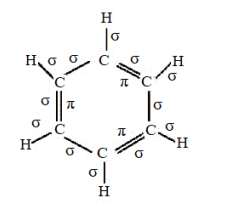There are $\operatorname{six} \mathrm{C}-\mathrm{C}$ sigma $\left(\sigma_{\mathrm{C}-\mathrm{C}}\right)$ bonds, $\operatorname{six} \mathrm{C}-\mathrm{H}$ sigma $\left(\sigma_{\mathrm{C}-\mathrm{H}}\right)$ bonds, and three $\mathrm{C}=\mathrm{C}$ Pi

$\left(\pi_{C-C}\right)$ resonating bonds in the given compound.

(ii) $\mathrm{C}_{6} \mathrm{H}_{12}$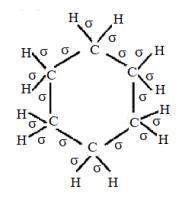There are six $\mathrm{C}-\mathrm{C}$ sigma $\left(\sigma_{\mathrm{C}-\mathrm{C}}\right)$ bonds and twelve $\mathrm{C}-\mathrm{H}$ sigma $\left(\sigma_{\mathrm{C}-\mathrm{H}}\right)$ bonds in the given compound.

(iii) $\mathrm{CH}_{2} \mathrm{Cl}_{2}$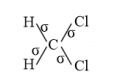There two $\mathrm{C}-\mathrm{H}$ sigma $\left(\sigma_{\mathrm{C}-\mathrm{H}}\right)$ bonds and two $\mathrm{C}-\mathrm{Cl}$ sigma $\left(\sigma_{\mathrm{C}-\mathrm{Cl}}\right)$ bonds in the given compound.

(iv) $\mathrm{CH}_{2}=\mathrm{C}=\mathrm{CH}_{2}$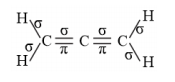There are two $\mathrm{C}-\mathrm{C}$ sigma $\left(\sigma_{\mathrm{C}-\mathrm{c}}\right)$ bonds, four $\mathrm{C}-\mathrm{H}$ sigma $\left(\sigma_{\mathrm{C}-\mathrm{H}}\right)$ bonds, and two $\mathrm{C}=\mathrm{C}$pi

$\left(\pi_{\mathrm{C}-\mathrm{C}}\right)$ bonds in the given compound.

(v) $\mathrm{CH}_{3} \mathrm{NO}_{2}$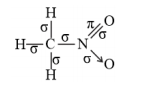There are three $\mathrm{C}-\mathrm{H}$ sigma $\left(\sigma_{\mathrm{C}-\mathrm{H}}\right)$ bonds, one $\mathrm{C}-\mathrm{N}$ sigma $\left(\sigma_{\mathrm{C}-\mathrm{N}}\right)$ bond, one $\mathrm{N}-\mathrm{O}$ sigma

$\left(\sigma_{N-0}\right)$ bond, and one $N=0$ pi $\left(\pi_{N-0}\right)$ bond in the given compound.

(vi) $\mathrm{HCONHCH}_{3}$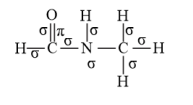There are two $\mathrm{C}-\mathrm{N}$ sigma $\left(\sigma_{\mathrm{C}-\mathrm{N}}\right)$ bonds, four $\mathrm{C}-\mathrm{H}$ sigma $\left(\sigma_{\mathrm{C}-\mathrm{H}}\right)$ bonds, one $\mathrm{N}-\mathrm{H}$ sigma

$\left(\sigma_{\mathrm{N}-\mathrm{H}}\right)$ bond, and one $\mathrm{C}=0$ pi $\left(\pi_{\mathrm{C}-\mathrm{o}}\right)$ bond in the given compound.

Question 3. Write bond-line formulas for: Isopropyl alcohol, 2,3-Dimethylbutanal, Heptan-4-one.

Solution: (a) Isopropyl alcohol

formulas of Isopropyl alcohol :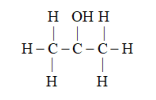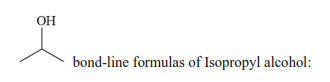(b) 2,3 -dimethyl butanal

formula of 2,3-Dimethylbutanal: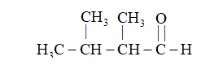$\Rightarrow$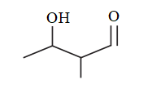bond line formula of 2,3 -Dimethylbutanal:

(C) Heptan- 4 -one

formulas for: Heptan-4-one: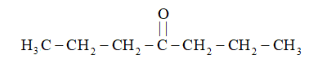$\Rightarrow$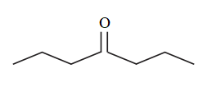bond line formulas of Heptan-4-one.

Question 4. Give the IUPAC names of the following compounds:

(a)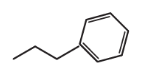(b)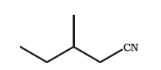(c)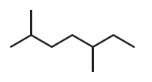(d)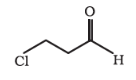(F) $\mathrm{Cl}_{2} \mathrm{CHCH}_{2} \mathrm{OH}$

Solution: $(\mathrm{A})$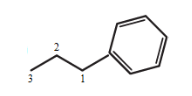3-phenyl propane

(B)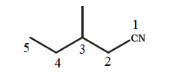2-methyl-1-cyanobutane

(C)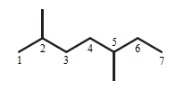2, 5- dimethyl heptane

(d)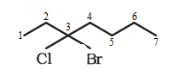3-bromo-3-chloroheptane

(e)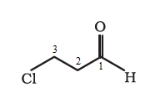3- chloropropanal

(f)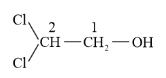2, 2-dichloroethan-1-ol

Question 5. Which of the following represents the correct IUPAC name for the compounds concerned?

(A) 2,2-Dimethylpentane or 2-Dimethylpentane

(B) $2,4,7$ -Trimethyloctane or $2,5,7$ -Trimethyloctane

(C) 2-Chloro-4-methylpentane or 4-Chloro-2-methylpentane

(D) But-3-yn-1-ol or But-4-ol-1-yne

Solution: (A) The prefix di in the IUPAC name indicates that two identical substituent $\left(-\mathrm{CH}_{3}\right)$ are present in the parent chain. Since two methyl groups are present in the $\mathrm{C}-2$ of the parent carbon chain of the given compound, the correct IPUAC name of the given compound is 2, 2-dimethylpentane.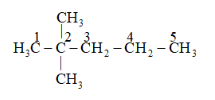(b) Locant number $2,4,7$ is lower than $2,5,7$. Hence, the IUPAC name of the given compound is $2,4,7$ -trimethyloctane.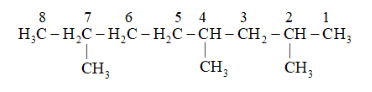(c) If the substituents are present in the equivalent position of the parent chain, then the lower number is given to the one that comes first in the name according to the alphabetical order and priorler group. Hence, the correct IUPAC name of the given compound is 2 -chloro- 4-methylpentane.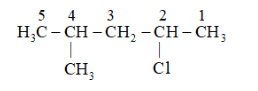(d) Two functional groups $-$ alcoholic and alkyne $-$ are present in the given compound. The principal functional group is the alcenolic group. Hence, the pareni chain will be suffixed with ol and get least number. The alkyne group is present in the $\mathrm{C}-3$ of the parent chain. Hence, the correct IUPAC name of the given compound is But-3 - yn $1-$ ol.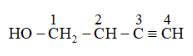Question 6. Draw formulas for the first five members of each homologous series beginning with the following compounds.

(A) $\mathrm{H}-\mathrm{COOH}$

(B) $\mathrm{CH}_{3} \mathrm{COCH}_{3}$

(C) $\mathrm{H}-\mathrm{CH}=\mathrm{CH}_{2}$

Solution: Homologous series : the series of compounds which have a difference of $-\mathrm{CH}_{2}$ or mass of $14 \mathrm{u}$.and the functional group should remain the same

The first five members of each homologous series beginning with the given compounds are shown as follows:

(a)

$\mathrm{H}-\mathrm{COOH}:$ Methanoic acid

$\mathrm{CH}_{3}-\mathrm{COOH}:$ Ethanoic acid

$\mathrm{CH}_{3}-\mathrm{CH}_{2}-\mathrm{COOH}:$ Propanoic acid

$\mathrm{CH}_{3}-\mathrm{CH}_{2}-\mathrm{CH}_{2}-$ COOH : Butanoic acid

$\mathrm{CH}_{3}-\mathrm{CH}_{2}-\mathrm{CH}_{2}-\mathrm{CH}_{2}-$ COOH : Pentanoic acid

(b)

$\mathrm{CH}_{3} \mathrm{COCH}_{3}:$ Propanone

$\mathrm{CH}_{3} \mathrm{COCH}_{2} \mathrm{CH}_{3}:$ Butanone

$\mathrm{CH}_{3} \mathrm{COCH}_{2} \mathrm{CH}_{2} \mathrm{CH}_{3}:$ Pentan-2-one

$\mathrm{CH}_{3} \mathrm{COCH}_{2} \mathrm{CH}_{2} \mathrm{CH}_{2} \mathrm{CH}_{3}:$ Hexan-2-one

$\mathrm{CH}_{3} \mathrm{CO} \mathrm{CH}_{2} \mathrm{CH}_{2} \mathrm{CH}_{2} \mathrm{CH}_{2} \mathrm{CH}_{3}:$ Heptan-2-one

(c)

$\mathrm{H}-\mathrm{CH}=\mathrm{CH}_{2}:$ Ethene

$\mathrm{CH}_{3}-\mathrm{CH}=\mathrm{CH}_{2}:$ Prop $-1$ -ene

$\mathrm{CH}_{3}-\mathrm{CH}_{2}-\mathrm{CH}=\mathrm{CH}_{2}:$ But-1-ene

$\mathrm{CH}_{3}-\mathrm{CH}_{2}-\mathrm{CH}_{2}-\mathrm{CH}=\mathrm{CH} 2:$ Pent- 1 -ene

$\mathrm{CH}_{3}-\mathrm{CH}_{2}-\mathrm{CH}_{2}-\mathrm{CH}_{2}-\mathrm{CH}=\mathrm{CH}_{2}:$ Hex-1-ene

Question 7. Give condensed and bond line structural formulas and identify the functional group(s) present, if any, for:

(A) 2,2,4-Trimethylpentane

(B) 2-Hydroxy-1,2,3-propanetricarboxylic acid

(C) Hexanedial

Solution: (a) 2, 2, 4 trimethylpentane

Condensed formula : $\left(\mathrm{CH}_{3}\right)_{2} \mathrm{CHCH}_{2} \mathrm{C}\left(\mathrm{CH}_{3}\right)_{3}$

Bond line formula: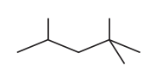(b) 2-hydroxy $-1,2,3$ -propanetricarboxylic acid

Condensed Formula: $(\mathrm{COOH}) \mathrm{CH}_{2} \mathrm{C}(\mathrm{OH})(\mathrm{COOH}) \mathrm{CH}_{2}(\mathrm{COOH})$

Bond line formula: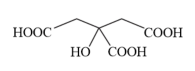The functional groups present in the given compound are carboxylic acid $(-\mathrm{COOH})$ and alcoholic $(-\mathrm{OH})$ groups.

(c) Hexanedial

Condensed Formula :

$(\mathrm{CHO})\left(\mathrm{CH}_{2}\right)_{4}(\mathrm{CHO})$

Bond line Formula :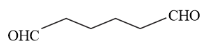The functional group present in the given compound is aldehyde ( $-\mathrm{CHO}$ ).

Question 8. Identify the functional groups in the following compounds

(a)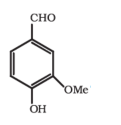Aldehyde (-CH0), Hydroxyl (-OH),

Methoxy $(-0 \mathrm{Me})$

$\mathrm{C}=\mathrm{C}$ double bond $\left(-{ }^{1}=\mathrm{C}-\right)$

(b)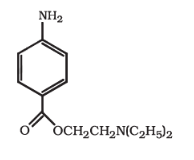Amino $\left(-\mathrm{NH}_{2}\right)$

Ester: RCOOR

Tertiary amine : $(\mathrm{R})_{3} \mathrm{~N}$

(c)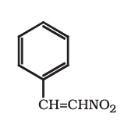Nitro $\left(-\mathrm{NO}_{2}\right)$

$\mathrm{C}=\mathrm{C}$ double bond (alkene) $(-\mathrm{C}=\mathrm{C}-)$

Question 9. Which of the two: $\mathrm{O}_{2} \mathrm{NCH}_{2} \mathrm{CH}_{2} \mathrm{O}^{-}$ or $\mathrm{CH}_{3} \mathrm{CH}_{2} \mathrm{O}^{-}$ is expected to be more stable and why?

Solution: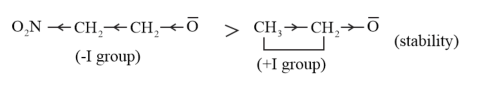$-\mathrm{NO}_{2}$ group is an electron-withdrawing group. It shows $-\mathrm{I}$ effect. By withdrawing the

electrons toward it, the $-\mathrm{NO}_{2}$ group decreases the negative charge density on the compound, thereby stabilising the negative charge. On the other hand, ethyl group is an electron-releasing group. It shows $+\mathrm{I}$ effect. This increases the negative charge on the compound, thereby destabilising the anion. Hence, $\mathrm{O}_{2} \mathrm{NCH}_{2} \mathrm{CH}_{2} \mathrm{O}^{-}$ is more stable than $\mathrm{CH}_{3} \mathrm{CH}_{2} \mathrm{O}^{-}$

Question 10. Explain why alkyl groups act as electron donors when attached to a $\pi$ system.

Solution: When an alkyl group is attached to a $\pi$ system, it acts as an electron-donor group by the

process of hyperconjugation. To understand this concept better, let us take the example

of propene.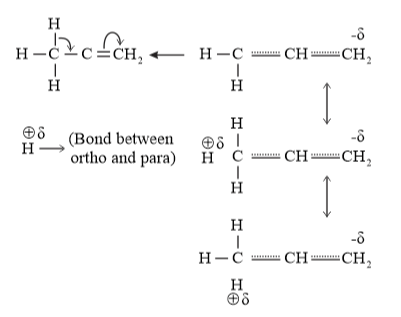In hyperconjugation, the sigma electrons of the $\mathrm{C}-\mathrm{H}$ bond of an alkyl group are delocalised. This group Is directly attached to an atom of an unsaturated system. The delocalisation occurs because of a partial overlap of a sp $^{3}-\mathrm{s}$ sigma bond orbital with an empty $\mathrm{p}$ orbital of the $\pi$ bond of an adjacent carbon atom.

The process of hyperconjugation in propene is shown as follows: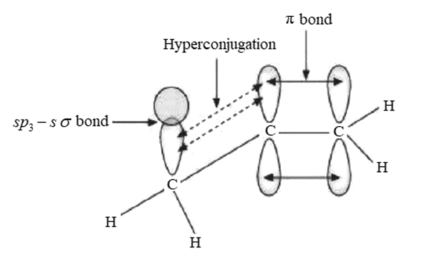This type of overlap leads to a delocalisation (also known as no-bond resonance) of the $\pi$ electrons, making the molecule more stavie.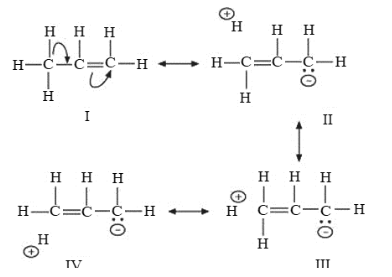Question 11. Draw the resonance structures for the following compounds. Show the electron shift using curved-arrow notation.

(A) $\mathrm{C}_{6} \mathrm{H}_{5} \mathrm{OH}$

(B) $\mathrm{C}_{6} \mathrm{H}_{5} \mathrm{NO}_{2}$

(C) $\mathrm{CH}_{3} \mathrm{CH}=\mathrm{CHCHO}$

(D) $\mathrm{C}_{6} \mathrm{H}_{5}-\mathrm{CHO}$

(e) $\mathrm{C}_{6} \mathrm{H}_{5}-{ }^{+} \mathrm{CH}_{2}$

(f) $\mathrm{CH}_{3} \mathrm{CH}=\mathrm{CHCH}_{2}^{+}$

Solution: (a) The structure of $\mathrm{C}_{6} \mathrm{H}_{5} \mathrm{OH}$ is: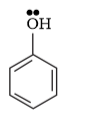The resonating structures of phenol are represented as: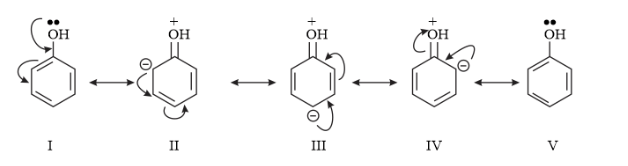(B) The structure of $\mathrm{C}_{6} \mathrm{H}_{5} \mathrm{NO}_{2}$ is: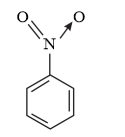The resonating structures of nitro benzene are represented as: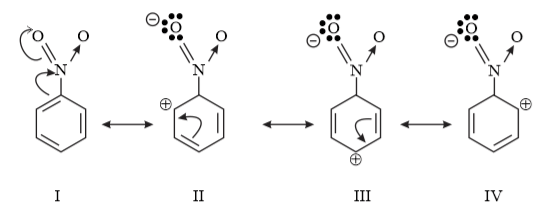(c) $\mathrm{CH}_{3} \mathrm{CH}=\mathrm{CH}-\mathrm{CHO}$

The resonating structures of the given compound are represented as: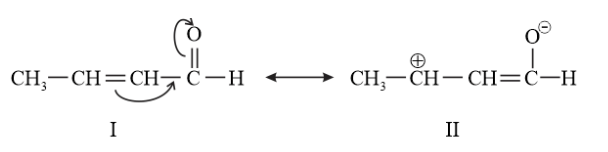(D) The structure of $\mathrm{C}_{6} \mathrm{H}_{5} \mathrm{CHO}$ is: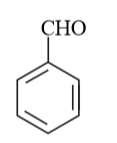The resonating structures of benzaldehyde are represented as: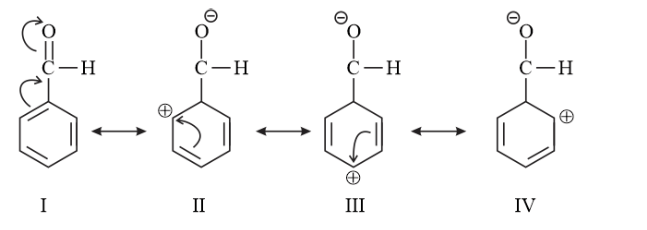(E) $\mathrm{C}_{6} \mathrm{H}_{5} \mathrm{CH}_{2}^{\oplus}$

The resonating structures of the given compound are: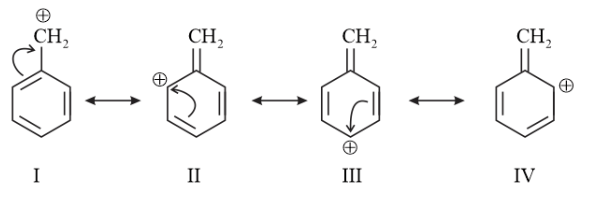(F) $\mathrm{CH}_{3} \mathrm{CH}=\mathrm{CHCH}_{2}^{\oplus}$

The resonating structures of the given compound are: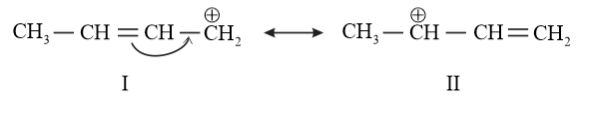Question 12. What are electrophiles and nucleophiles? Explain with examples.

Solution: Electro means electron and phile means loving so electrophile si electron loving. In other words, an electron-deficient reagent is called an electrophile $\left(\mathrm{E}^{+}\right)$.since Electrophiles are electron deficient so they can accept electron pair. Some positively charged species (carbocation) and octet deficient species are electrophiles

examples of electrophiles.

Carbocations $\left(\mathrm{CH}_{3} \mathrm{CH}_{2}^{+}\right), \mathrm{Cl}^{+}, \mathrm{Br}^{+}, \mathrm{I}^{+}$ has 6 electrons in valance shell

neutral molecules like BF $_{3}, \mathrm{AlCl}_{3}$ has 6 electrons in valance shell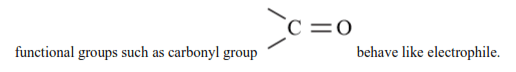nucleophile (Nu:) : Nucleo means nucleus and phile means loving so nucleophile is nucleus loving. In other words, an electron-rich reagent is called a nucleophile (nu $^{-}$ ).A nucleophile is a reagent that brings an electron pair or negative charge density.

For example negatively charged species like $\mathrm{OH}^{-}, \mathrm{NC}^{-}$, carbanions $\left.\mathrm{R}_{3} \mathrm{C}^{-}\right), \mathrm{F}^{-}, \mathrm{Cl}^{-}, \mathrm{I}^{-}, \mathrm{Br}^{-}, \mathrm{N}_{3}^{-}$, etc.

Neutral molecules such as $\mathrm{H}_{2} \mathrm{O}$ and ammonia also acts as nucleophiles because of the presence of a lone pair.

Question 13. Identify the reagents shown in bold in the following equations as nucleophiles or electrophiles:

(A) $\mathrm{CH}_{3} \mathrm{COOH}+\mathrm{HO}^{-} \rightarrow \mathrm{CH}_{3} \mathrm{COO}^{-}+\mathrm{H}_{2} \mathrm{O}$

(B) $\mathrm{CH}_{3} \mathrm{COCH}_{3}+\overline{\mathrm{C}} \mathrm{N} \rightarrow\left(\mathrm{CH}_{3}\right)_{2} \mathrm{C}(\mathrm{CN})(\mathrm{OH})$

(C) $\mathrm{C}_{6} \mathrm{H}_{6}+\mathrm{CH}_{3}^{+} \mathrm{C}=0 \rightarrow \mathrm{C}_{6} \mathrm{H}_{5} \mathrm{COCH}_{3}$

Solution: Electrophiles are electron-deficient species and can receive an electron pair. On the other

hand, nucleophiles are electron-rich species and can donate their electrons.

(A) $\mathrm{CH}_{3} \mathrm{COOH}+\mathrm{HO}^{-} \rightarrow \mathrm{CH}_{3} \mathrm{COO}^{-}+\mathrm{H}_{2} \mathrm{O}$

Here, $\mathrm{HO}^{-}$ acts as a nucleophile as it is an electron-rich species, i.e., it is a nucleus seeking

species.

(B) $\mathrm{CH}_{3} \mathrm{COCH}_{3}+\overline{\mathrm{C}} \mathrm{N} \rightarrow\left(\mathrm{CH}_{3}\right)_{2} \mathrm{C}(\mathrm{CN})+(\mathrm{OH})$

Here, ${ }^{-} \mathrm{CN}$ acts as a nucleophile as it is an electron-rich species, it will attack at nucleus or electron-deficient side

(C) $\mathrm{C}_{6} \mathrm{H}_{5}+\mathrm{CH}_{3}^{+} \mathrm{CO} \rightarrow \mathrm{C}_{6} \mathrm{H}_{5} \mathrm{COCH}_{3}$

Here, $\mathrm{CH}_{3}^{+} \mathrm{CO}$ acts as an electrophile as it is an electron-deficient species or it attack at electron rich side.

Question 14. Classify the following reactions in one of the reaction type studied in this unit.

(A) $\mathrm{CH}_{3} \mathrm{CH}_{2} \mathrm{Br}+\mathrm{HS}^{-} \rightarrow \mathrm{CH}_{3} \mathrm{CH}_{2} \mathrm{SH}+\mathrm{Br}^{-}$

(B) $\left(\mathrm{CH}_{3}\right)_{2} \mathrm{C}=\mathrm{CH}_{2}+\mathrm{HCl} \rightarrow\left(\mathrm{CH}_{3}\right)_{2} \mathrm{ClC}-\mathrm{CH}_{3}$

(C) $\mathrm{CH}_{3} \mathrm{CH}_{2} \mathrm{Br}+\mathrm{HO}^{-} \rightarrow \mathrm{CH}_{2}=\mathrm{CH}_{2}+\mathrm{H}_{2} \mathrm{O}+\mathrm{Br}^{-}$

(D) $\left(\mathrm{CH}_{3}\right)_{3} \mathrm{C}-\mathrm{CH}_{2} \mathrm{OH}+\mathrm{HBr} \rightarrow\left(\mathrm{CH}_{3}\right)_{2} \mathrm{CBrCH}_{2} \mathrm{CH}_{2} \mathrm{CH}_{3}+\mathrm{H}_{2} \mathrm{O}$

Solution: (A) $\mathrm{CH}_{3} \mathrm{CH}_{2} \mathrm{Br}+\mathrm{HS}^{-} \rightarrow \mathrm{CH}_{3} \mathrm{CH}_{2} \mathrm{SH}+\mathrm{Br}^{-}$

It is an example of nucleophilic substitution reaction as in this reaction the bromine group in is leaving group and -Br group is substituted by the $-\mathrm{SH}$ group.

(B) $\left(\mathrm{CH}_{3}\right)_{2} \mathrm{C}=\mathrm{CH}_{2}+\mathrm{HCl} \rightarrow\left(\mathrm{CH}_{3}\right)_{2} \mathrm{ClC}-\mathrm{CH}_{3}$

It is an example of electrophilic addition reaction as in this reaction two reactant molecules combine to form product.

(D) $\left(\mathrm{CH}_{3}\right)_{3} \mathrm{C}-\mathrm{CH}_{2} \mathrm{OH}+\mathrm{HBr}$

$\rightarrow\left(\mathrm{CH}_{3}\right)_{2} \mathrm{CBrCH}_{2} \mathrm{CH}_{2} \mathrm{CH}_{3}+\mathrm{H}_{2} \mathrm{O}$

In this reaction, substitution takes place, $-\mathrm{OH}$ group is replaced with -Br group and it followed by a rearrangement of atoms and groups of atoms.

Question15. What is the relationship between the members of following pairs of structures? Are they structural or geometrical isomers or resonance contributors?

(a)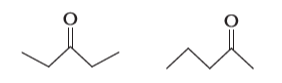(c)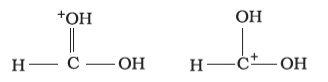Solution: (A) Compounds having the same molecular formula but with different structures are called structural isomers. The given compounds have the same function group (ketone) but they differ in the position.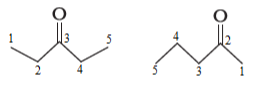(B) Compounds having the same molecular formula, the same connectivity of atoms and their arrangement in space is different .in the given compounds the restricted rotation present du to double bond and different groups attached with carbon, so they are called geometrical isomers.

Priority of group $\mathrm{D}>\mathrm{H}$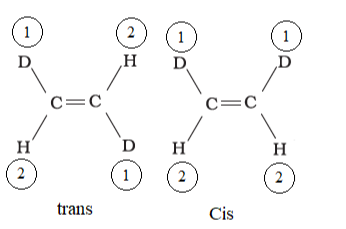In structures I and II, the relative position of Deuterium (D) and hydrogen (H) in space are different. Hence, the given pairs represent geometrical isomers.

(C) The given structures are canonical structures or contributing structures. They are hypothetical and individually do not represent any real molecule. Hence, the given pair

represents resonance structures, called resonance isomers.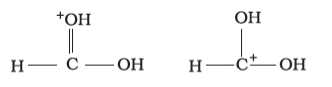Question 16. For the following bond cleavages, use curved-arrows to show the electron flow and classify each as homolysis or heterolysis. Identify reactive intermediate produced as free radical, carbocation and carbanion.

(a)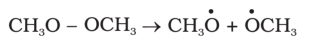(b)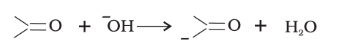(c)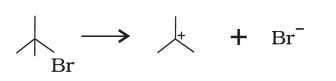(d)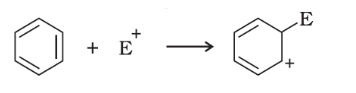Solution: (a) $\mathrm{CH}_{3} \mathrm{O}-\mathrm{OCH}_{3} \rightarrow \mathrm{CH}_{3} \dot{\mathrm{O}}+\dot{\mathrm{O}} \mathrm{CH}_{3}$

It is an example of homolytic cleavage as one of the shared pair in a covalent bond goes with the bonded atom because there is no electronegativity difference. The reaction intermediate formed is a free radical.

The bond cleavage using half curved-arrows to show the electron flow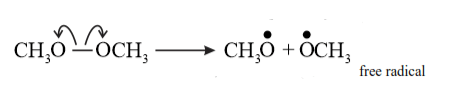(b)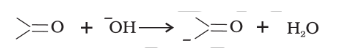In this reaction base $\left(\mathrm{OH}^{-}\right)$ snatched the acidic hydrogen $.$ acidic hydrogen leave as $\left(\mathrm{H}^{+}\right)$

There is an electronegativity difference between carbon and hydrogen so it is an example of heterolytic cleavage as the bond breaks in such a manner that the shared pair of electrons remains with the carbon of propanone. The reaction intermediate formed is carbanion

The bond cleavage using curved-arrows to show the electron flow of the given reaction can be represented as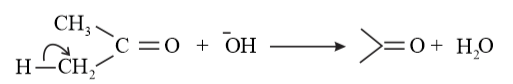(c)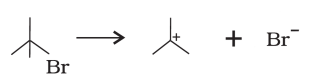It is an example of heterolytic cleavage because of electronegativity difference in between Carbon and Bromine.as the bond breaks in such a manner that the shared pair of electrons remains with the bromine ion and carbon has electron deficiency so reaction intermediate formed is a carbocation.

The bond cleavage using curved-arrows to show the electron flow of the given reaction

(d)It is a heterolytic cleavage as the bond breaks in such a manner that the shared pair of electrons remains with one of the fragments. The intermediate formed is a carbocation.

Question 17. Explain the terms Inductive and Electromeric effects. Which electron displacement effect explains the following correct orders of acidity of the carboxylic acids?

(A) $\mathrm{Cl}_{3} \mathrm{CCOOH}>\mathrm{Cl}_{2} \mathrm{CHCOOH}>\mathrm{ClCH}_{2} \mathrm{COOH}$

(B) $\mathrm{CH}_{3} \mathrm{CH}_{2} \mathrm{COOH}>\left(\mathrm{CH}_{3}\right)_{2} \mathrm{CHCOOH}>\left(\mathrm{CH}_{3}\right)_{3} \mathrm{C} . \mathrm{COOH}$

Solution: Inductive effect is a permanent displacement of sigma $(\sigma)$ electrons along a saturated chain, whenever an electron-withdrawing or electron-donating group is present, is called the inductive effect. It occurs due to electronegativity of the difference of atoms

It is a feeble effect and as the distance increases the strength of the inductive effect decreases

Inductive effect $\propto \frac{1}{\text { distance }}$

The inductive effect is two types $+\mathrm{I}$ effect or $-\mathrm{I}$ effect.

$-$ I effect: When an atom or group attract electrons towards itself more strongly than hydrogen

For example,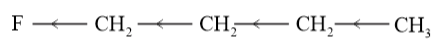$+$ I effect: When an atom or group attract electrons towards itself less strongly than hydrogen.

For example,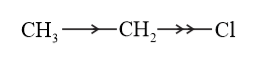Electrometric effect

It involves the complete transfer of the shared pair of $\pi$ electrons to either of the two atoms linked by multiple bonds in the presence of an attacking agent. For example,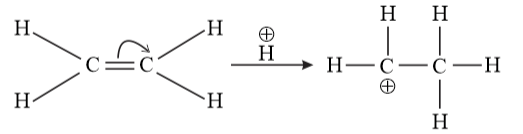Electrometric effect could be $+\mathrm{E}$ effect or $-\mathrm{E}$ effect.

$+\mathrm{E}$ effect: When the electrons are transferred towards the attacking reagent

-E effect: When the electrons are transferred away from the attacking reagent

(a) $\mathrm{Cl}_{3} \mathrm{CCOOH}>\mathrm{Cl}_{2} \mathrm{CHCOOH}>\mathrm{ClCH}_{2} \mathrm{COOH}$

Acidic strength $\propto$ stability of conjugate base (Anion)

Stability of conjugate base or anion $\propto \frac{1}{\text { electron density }}$

The order of acidity can be explained on the basis of Inductive effect (-I effect). As the number of chlorine atoms increases, the $-\mathrm{I}$ effect increases. With the increase in $-\mathrm{I}$ effect, the acid strength also increases accordingly.

Conjugate base stability or acidic strength order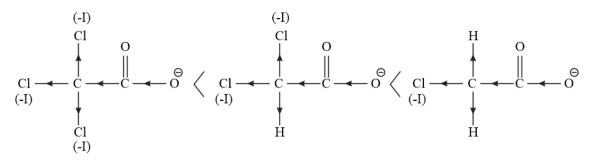Electron density order.

(B) $\mathrm{CH}_{3} \mathrm{CH}_{2} \mathrm{COOH}>\left(\mathrm{CH}_{3}\right)_{2} \mathrm{CHCOOH}>\left(\mathrm{CH}_{3}\right)_{3} \mathrm{C} . \mathrm{COOH}$

Acidic strength $\propto$ stability of conjugate base (Anion)

Stability of conjugate base or anion $\propto \frac{1}{\text { electron density }}$

The order of acidity can be explained on the basis of inductive effect (+I effect). As the number of alkyl groups increases, the $+$ I effect also increases. With the increase in $+\mathrm{I}$ effect, the acid strength also increases accordingly.

Stability of anion or conjugate base order.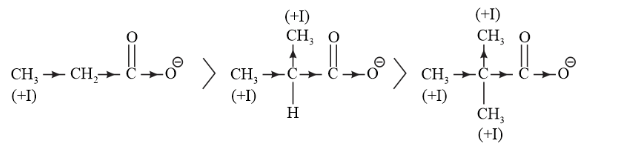Electron density order.

Question 18. Give a brief description of the principles of the following techniques taking an example in each case.

(A) Crystallisation

(B) Distillation

(C) Chromatography

Solution: (a) Crystallisation

Crystallisation techniques is used for the purification of solid organic compounds.

Principle: used : It is based on the difference in the solubilities of the compound and the impurities in a suitable solvent. The impure compound is dissolved in a solvent in which it is sparingly soluble at room temperature but appreciably soluble at higher temperature. The solution is concentrated to get a nearly saturated solution. On cooling the solution, pure compound crystallises out and is removed by filtration.

The filtrate (mother liquor) contains impurities and small quantity of the compound. If the compound is highly soluble in one solvent and very little soluble in another solvent, crystallisation can be satisfactorily carried out in a mixture of these solvents. Impurities, which impart colour to the solution are removed by adsorbing over activated charcoal. Repeated crystallization becomes necessary for the purification of compounds containing impurities of comparable solubilities.

For example, pure aspirin is obtained by recrystallising crude aspirin. Approximately $2-$ $4 \mathrm{~g}$ of crude aspirin is dissolved in about $20 \mathrm{~mL}$ of ethyl alcohol. The solution is heated

(if necessary) to ensure complete dissolution. The solution is then left undisturbed until some crystals start to separate out. The crystals are then filtered and dried.

(B) Distillation : used to separate volatile liquids from non-volatile impurities or a mixture

of those liquids that have a sufficient difference in their boiling points.

Principle: It is based on the fact that liquids having different boiling points vapourise at different temperatures. The vapours are then cooled and the liquids so formed are collected separately.

For example, a mixture of chloroform (b. $p=334 \mathrm{~K}$ ) and aniline (b. $\mathrm{p}=457 \mathrm{~K}$ ) can be

separated by the method of distillation. The mixture is taken in a round bottom flask fitted

with a condenser. It is then heated. Chloroform, being more volatile, vaporizes first and

passes into the condenser. In the condenser, the vapours condense and chloroform trickles

down. In the round bottom flask, aniline is left behind.

(C) Chromatography : useful methods for the separation and purification of organic compounds.

Principle: It is based on the difference in movement of individual components of a mixture

through the stationary phase under the influence of mobile phase.

For example, a mixture of red and blue ink can be separated by chromatography. A drop

of the mixture is placed on the chromatogram. The component of the ink, which is less

adsorbed on the chromatogram, moves with the mobile phase while the less adsorbed

component remains almost stationary.

Question 19. Describe the method, which can be used to separate two compounds with different solubilities in a solvent.

Solution:

Fractional crystallisation is the method used for separating two compounds with different

solubilities in a solvent.

The process of fractional crystallisation is carried out in four steps.

Step 1 - Preparation of the solution: The powdered mixture is taken in a flask and the solvent is added to it slowly and stirred simultaneously. The solvent is added till the solute is just dissolved in the solvent. This saturated solution is then heated.

Step 2 - Filtration of the solution: The hot saturated solution is then filtered through a filter paper in a China dish.

Step 3- Fractional crystallisation: The solution in the China dish is now allowed to cool. The less soluble compound crystallises first, while the more soluble compound remains in the solution. After separating these crystals from the mother liquor, the latter is concentrated once again. The hot solution is allowed to cool and consequently, the crystals of the more soluble compound are obtained.

Step 4: Isolation and drying: These crystals are separated from the mother liquor by filtration. Finally, the crystals are dried.

Question 20. What is the difference between distillation, distillation under reduced pressure and steam distillation?

Solution: The differences among distillation, distillation under reduced pressure, and steam distillation are given in the following table.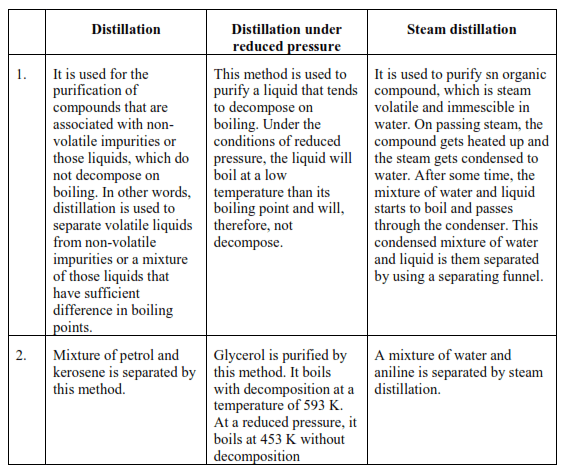The differences among distillation, distillation under reduced pressure, and steam distillation are given in the following table.

Question 21. Discuss the chemistry of Lassaigne's test.

Solution: Lassaigne's test

This test is used to detect the presence of nitrogen, sulphur, halogens, and phosphorous in an organic compound. These elements are present in the covalent form in an organic compound. These are converted into the ionic form by fusing the compound with sodium metal.

$\mathrm{Na}+\mathrm{C}+\mathrm{N} \stackrel{\Delta}{\longrightarrow} \mathrm{NaCN}$

$2 \mathrm{Na}+\mathrm{S} \stackrel{\Delta}{\longrightarrow} \mathrm{Na}_{2} \mathrm{~S}$

$\mathrm{Na}+\mathrm{X} \stackrel{\Delta}{\longrightarrow} \mathrm{NaX}$

$(\mathrm{X}=\mathrm{Cl}, \mathrm{Br}, \mathrm{I})$

The cyanide, sulphide, and halide of sodium formed are extracted from the fused mass by

boiling it in distilled water. The extract so obtained is called Lassaigne's extract.

(a) Test for nitrogen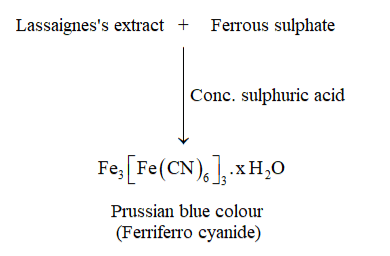In the Lassaigne's test for nitrogen in an organic compound, the sodium fusion extract is

boiled with iron (II) sulphate and then acidified with concentrated sulphuric acid. In the process, sodium

cyanide first reacts with iron (II) sulphate and forms sodium hexacyanoferrate (II). which is Prussian blue in colour Then,on heating with sulphuric acid, some iron (II) gets oxidised to form iron (III)

$6 \mathrm{CN}^{-}+\mathrm{Fe}^{2+} \longrightarrow\left[\mathrm{Fe}(\mathrm{CN})_{6}\right]^{4-}$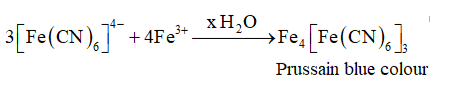(B) Test for sulphur

(i) Lassaigne's extract $+$ Lead acetate $\stackrel{\text { acetic acid }}{\longrightarrow}$ Black precipitate.

In the Lassaigne's test for sulphur in an organic compound, the sodium fusion extract is

sulphide, which is black in colour, indicates the presence of sulphur in the compound.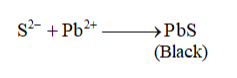or

Lassaigne's extract $+$ Sodium nitroprusside $\rightarrow$ Violet colour.

The sodium fusion extract is treated with sodium nitroprusside. Appearance of violet colour

Also indicates the presence of sulphur in the compound.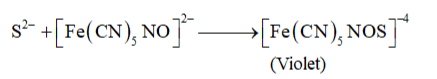If in an organic compound, both nitrogen and sulphur are present, then instead of $\mathrm{NaCN}$,

formation of NaSCN takes place.

$\mathrm{Na}+\mathrm{C}+\mathrm{N}+\mathrm{S} \rightarrow \mathrm{NaSCN}$

This NaSCN (sodium thiocyanate) gives a blood red colour. Prussian colour is not formed due to the absence of free cyanide ions.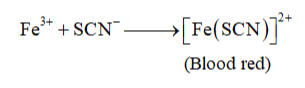(C) Test for halogens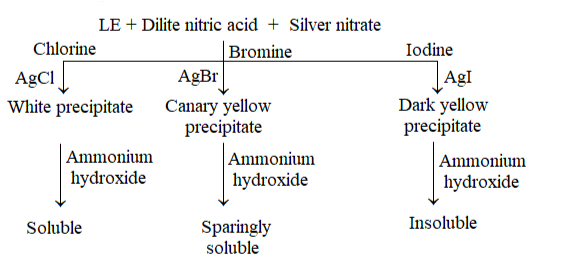In the Lassaigne's test for halogens in an organic compound, the sodium fusion extract is

acidified with nitric acid and then treated with silver nitrate.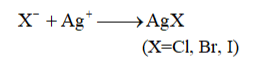If nitrogen and sulphur both are present in the organic compound, then the Lassaigne's

extract is boiled to expel nitrogen and sulphur, which would otherwise interfere in the test

for halogens.

Question 22. Differentiate between the principle of estimation of nitrogen in an organic

compound by

(i) Dumas method and

(ii) Kjeldahl's method.

Solution:

In Dumas method, a known quantity of nitrogen containing organic compound is heated

strongly with excess of copper oxide in an atmosphere of carbon dioxide to produce free

nitrogen in addition to carbon dioxide and water. The chemical equation involved in the

process can be represented as

$\mathrm{CxHyNz}+(2 \mathrm{x}+\mathrm{y} / 2) \mathrm{Cu} \mathrm{O} \rightarrow \mathrm{x} \mathrm{CO}_{2}$

$+\mathrm{y} / 2 \mathrm{H}_{2} \mathrm{O}+\mathrm{z} / 2 \mathrm{~N}_{2}+(2 \mathrm{x}+\mathrm{y} / 2) \mathrm{Cu}$

The traces of nitrogen oxides can also be produced in the reaction, which can be reduced

to dinitrogen by passing the gaseous mixture over a heated copper gauge. The dinitrogen

produced is collected over an aqueous solution of potassium hydroxide. The volume of nitrogen produced is then measured at room temperature and atmospheric pressure.

(ii) Kjeldahl's method: a known quantity of nitrogen containing organic compound is heated with concentrated sulphuric acid. The nitrogen present in the compound is quantitatively converted into ammonium sulphate. It is then distilled with excess of sodium hydroxide. The ammonia evolved during this process is passed into a known volume of $\mathrm{H}_{2} \mathrm{SO}_{4} .$ The chemical equations involved in the process are

Organic compound $\stackrel{\text { Conc } \mathrm{H}_{2} \mathrm{SO}_{4}}{\longrightarrow}\left(\mathrm{NH}_{4}\right)_{2} \mathrm{SO}_{4}$

$\left(\mathrm{NH}_{4}\right)_{2} \mathrm{SO}_{4}+2 \mathrm{NaOH} \longrightarrow \mathrm{Na}_{2} \mathrm{SO}_{4}+2 \mathrm{NH}_{3}+2 \mathrm{H}_{2} \mathrm{O}$

$2 \mathrm{NH}_{3}+\mathrm{H}_{2} \mathrm{SO}_{4} \longrightarrow\left(\mathrm{NH}_{4}\right)_{2} \mathrm{SO}_{4}$

The acid that is left unused is estimated by volumetric analysis (titrating it against a standard alkali) and the amount of ammonia produced can be determined. Thus, the percentage of nitrogen

in the compound can be estimated. This method cannot be applied to the compounds, in which nitrogen is present in a ring structure, and also not applicable to compounds containing nitro and azo groups.

Question 23. Discuss the principle of estimation of halogens, sulphur and phosphorus present in an organic compound.

Solution: Estimation of halogens

Halogens are estimated by the Carius method. In this method, a known quantity of organic compound is heated with fuming nitric acid in the presence of silver nitrate, contained in a hard glass tube called the Carius tube, taken in a furnace. Carbon and hydrogen that are present in the compound are oxidized to form $\mathrm{CO}_{2}$ and $\mathrm{H}_{2} \mathrm{O}$ respectively and the halogen present in the compound is converted to the form of $\mathrm{AgX}$.

This $\mathrm{AgX}$ is then filtered, washed, dried, and weighed.

Let the mass of organic compound be $\mathrm{m} \mathrm{g}$.

Mass of $\mathrm{AgX}$ formed $=\mathrm{m}_{1} \mathrm{~g}$

1 mol of $A g X$ contains 1 mol of $X$

Therefore,

Mass of halogen in $\mathrm{m}_{1} \mathrm{~g}$ of $\mathrm{AgX}=\frac{\text { Atomic mass of } \mathrm{X} \times \mathrm{m}_{1} \mathrm{~g}}{\text { Molecular mass of } \mathrm{AgX}}$

Thus, $\%$ of halogen will be $=\frac{\text { Atomic mass of } \mathrm{X} \times \mathrm{m}_{1} \times 100}{\text { Molecular mass of } \mathrm{AgX} \times \mathrm{m}}$

Estimation of Sulphur

In this method, a known quantity of organic compound is heated with either fuming nitric acid or sodium peroxide in a hard glass tube called the Carius tube. Sulphur, present in the compound, is oxidized to form sulphuric acid. On addition of excess of barium chloride to it, the precipitation of barium sulphate takes place. This precipitate is then filtered, washed, dried, and weighed.

Let the mass of organic compound be $\mathrm{mg}$.

Mass of $\mathrm{BaSO}_{4}$ formed $=\mathrm{m}_{1} \mathrm{~g}$

$1 \mathrm{~mol}$ of $\mathrm{BaSO}_{4}=233 \mathrm{~g} \mathrm{BaSO}_{4}=32 \mathrm{~g}$ of Sulphur

Therefore, $\mathrm{m}_{1} \mathrm{~g}$ of $\mathrm{BaSO}_{4}$ contains $\frac{32 \times \mathrm{m}_{1}}{233} \mathrm{~g}$ of Sulphur.

Thus, percentage of Sulphur $=\frac{32 \times \mathrm{m}_{1} \times 100}{233 \times \mathrm{m}}$.

Estimation of phosphorus

In this method, a known quantity of organic compound is heated with fuming nitric acid. Phosphorus, present in the compound, is oxidized to form phosphoric acid. By adding ammonia and ammonium molybdate to the solution, phosphorus can be precipitated as ammonium phosphomolybdate.

Phosphorus can also be estimated by precipitating it as $\mathrm{MgNH}_{4} \mathrm{PO}_{4}$ by adding magnesia mixture, which on ignition yields $\mathrm{Mg}_{2} \mathrm{P}_{2} \mathrm{O}_{7}$.

Let the mass of organic compound be $\mathrm{m} \mathrm{g}$.

Mass of ammonium phosphomolybdate formed $=\mathrm{m}_{1} \mathrm{~g}$

Molar mass of ammonium phosphomolybdate $=1877 \mathrm{~g}$

Thus, percentage of phosphorous $=\frac{31 \times \mathrm{m}_{1} \times 100}{1877 \times \mathrm{m}} \%$

If $\mathrm{P}$ is estimated as $\mathrm{Mg}_{2} \mathrm{P}_{2} \mathrm{O}_{7}$

Then, percentage of phosphorous $=\frac{62 \times \mathrm{m}_{1} \times 100}{222 \times \mathrm{m}} \%$

Question 24. Explain the principle of paper chromatography.

Solution: In paper chromatography, chromatography paper is used. This paper contains water trapped in it, which acts as the stationary phase. On the base of this chromatography paper, the solution of the mixture is spotted. The paper strip is then suspended in a suitable solvent, which acts as the mobile phase. This solvent rises up the ehromatography paper by eapillary aetio.n and in the proeedure, it flows over the spot. The components are selectively retained on the paper (according to their differing partition in these two phases). The spots of different components travel with the mobile phase to different heights. The paper so obtained (shown in the given figure) is known as a chromatogram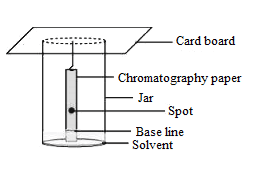Question 25. Why is nitric acid added to sodium extract before adding silver nitrate for testing halogens?

Solution: While testing the Lassaigne's extract for the presence of halogens, it is first boiled with dilute nitric acid. This is done to decompose $\mathrm{NaCN}$ to $\mathrm{HCN}$ and $\mathrm{Na}_{2} \mathrm{~S}$ to $\mathrm{H}_{2} \mathrm{~S}$ and to expel these gases. That is, if any nitrogen and sulphur are present in the form of $\mathrm{NaCN}$ and $\mathrm{Na}_{2} \mathrm{~S}$, then they are removed. The chemical equations involved in the reaction are represented as

$\mathrm{NaCN}+\mathrm{HNO}_{3} \rightarrow \mathrm{NaNO}_{3}+\mathrm{HCN}$

$\mathrm{Na}_{2} \mathrm{~S}+2 \mathrm{HNO}_{3} \rightarrow 2 \mathrm{NaNO}_{3}+\mathrm{H}_{2} \mathrm{~S}$

Question 26. Explain the reason for the fusion of an organic compound with metallic sodium for testing nitrogen, sulphur and halogens.

solufion: Nitrogen, sulphur, and halogens are covalently bonded in organic compounds. For their detection, they have to be first converted to ionic form. This is done by fusing the organic compound with sodium metal. This is called "Lassaigne's test". The chemical equations involved in the test are

$\mathrm{Na}+\mathrm{C}+\mathrm{N} \rightarrow \mathrm{NaCN}$

$\mathrm{Na}+\mathrm{S}+\mathrm{C}+\mathrm{N} \rightarrow \mathrm{NaSCN}$

$2 \mathrm{Na}+\mathrm{S} \rightarrow \mathrm{Na}_{2} \mathrm{~S}$

$\mathrm{Na}+\mathrm{X} \rightarrow \mathrm{NaX}$

$(\mathrm{X}=\mathrm{Cl}, \mathrm{Br}, \mathrm{I})$

Carbon, nitrogen, sulphur, and halogen come from organic compounds.

Question 27. Name a suitable technique of separation of the components from a mixture of calcium sulphate and camphor.

solufon: The process of sublimation is used to separate a mixture of camphor and calcium sulphate. In this process, the sublimable compound changes from solid to vapour state without passing through the

liquid state. Camphor is a sublimable compound and calcium sulphate is a nonsublimable solid. Hence, on heating, camphor will sublime while calcium sulphate will be left behind.

Question28. Explain, why an organic liquid vaporises at a temperature below its boiling point in its steam distillation?

Solution: In steam distillation, the organic liquid starts to boil when the sum of vapour pressure due to the organic liquid $\left(\mathrm{p}_{1}\right)$ and the vapour pressure due to water $\left(\mathrm{p}_{2}\right)$ becomes equal to atmospheric pressure $(\mathrm{p})$, that is, $\mathrm{p}=\mathrm{p}_{1}+\mathrm{p}_{2}$

Since $\mathrm{p}_{1}<\mathrm{p}_{2}$, organic liquid will vapourise at a lower temperature than its boiling point.

Question 29. Will CCl $_{4}$ give white precipitate of $\mathrm{AgCl}$ on heating it with silver nitrate? Give reason for your answer.

Sulution: Halogen containing ionic compound $+\mathrm{AgNO}_{3} \rightarrow \mathrm{AgCl}$ (white ppt)

Halogen containing Organic compound $\mathrm{AgNO}_{3} \rightarrow$ no reaction

In $\mathrm{CCl}_{4}$ the chlorine atoms are covalently bonded with the to carbon so it will not give the white precipitate of $\mathrm{AgCl}$ on heating it with silver nitrate. $\mathrm{Cl}$ atom should

be present in ionic formi the compound, it is necessary to prepare the Lassaigne's extract of $\mathrm{CCl}_{4}$.

Question 30. Why is a solution of potassium hydroxide used to absorb carbon dioxide evolved during the estimation of carbon present in an organic compound?

Solution: Carbon dioxide is acidic in nature and potassium hydroxide is a strong base.so acid-base reaction takes place .carbon dioxide reacts with potassium hydroxide to form potassium carbonate and water

$2 \mathrm{KOH}+\mathrm{CO}_{2} \rightarrow \mathrm{K}_{2} \mathrm{CO}_{3}+\mathrm{H}_{2} \mathrm{O}$

Thus, the mass of the U-tube containing KOH increases. This increase in the mass of $\mathrm{U}-$ tube gives the mass of $\mathrm{CO}_{2}$ produced. From its mass, the percentage of carbon in the organic compound can be estimated.

Question 31. Why is it necessary to use acetic acid and not sulphuric acid for acidification of sodium extract for testing sulphur by lead acetate test?

Solution: $\mathrm{CH}_{3} \mathrm{COOH}$ (acetic acid ) $\rightarrow \mathrm{CH}_{3} \mathrm{COO}^{-}+\mathrm{H}^{+}$

$\mathrm{H}_{2} \mathrm{SO}_{4}$ (sulphuric acid) $\rightarrow \mathrm{HSO}_{4}+\mathrm{H}^{+}$

Although the addition of sulphuric acid will precipitate lead sulphate, the addition of acetic acid will ensure a complete precipitation of sulphur in the form of lead sulphate due to common ion effect. Hence, it is necessary to use acetic acid for acidification of sodium extract for testing sulphur by lead acetate test.

Question 32. An organic compound contains $69 \%$ carbon and $4.8 \%$ hydrogen, the remainder being oxygen. Calculate the masses of carbon dioxide and water produced when $0.20 \mathrm{~g}$ of this substance is subjected to complete combustion.

Solution: Given: weight of comound $=100 \mathrm{gm}$

We know : Molecular mass of carbon dioxide, $\mathrm{CO}_{2}=44 \mathrm{~g}$

Percentage of carbon in organic compound $=69 \%$

That is, $100 \mathrm{~g}$ of organic compound contains $69 \mathrm{~g}$ of carbon.

$\therefore 0.2 \mathrm{~g}$ of organic compound will contain $=\frac{69 \times 0.2}{100}=0.138 \mathrm{~g}$ of $\mathrm{C}$

Molecular mass of carbon dioxide $\left(\mathrm{CO}_{2}\right)=12+2 \times 16=44 \mathrm{~g}$

That is, $12 \mathrm{~g}$ of carbon is contained in $44 \mathrm{~g}$ of $\mathrm{CO}_{2}$.

Therefore, $0.138 \mathrm{~g}$ of carbon will be contained in $\frac{44 \times 0.138}{12}=0.506 \mathrm{~g}$ of $\mathrm{CO}_{2}$

Thus, $0.506 \mathrm{~g}$ of $\mathrm{CO}_{2}$ will be produced on complete combustion of $0.2 \mathrm{~g}$ of organic compound.

Percentage of hydrogen in organic compound is $4.8$.

i.e., $100 \mathrm{~g}$ of organic compound contains $4.8 \mathrm{~g}$ of hydrogen.

Therefore, $0.2 \mathrm{~g}$ of the organic compound will contain $\frac{4.8 \times 0.2}{100}=0.0096 \mathrm{~g} \mathrm{H}$

It is known that the molecular mass of water $\left(\mathrm{H}_{2} \mathrm{O}\right)$ is $18 \mathrm{~g}$.

Thus, $2 \mathrm{~g}$ of hydrogen is contained in $18 \mathrm{~g}$ of water.

$\therefore 0.0096 \mathrm{~g}$ of hydrogen will be contained in of water $\frac{18 \times 0.0096}{2}=0.0864 \mathrm{~g}$ of water.

Thus, $0.0864 \mathrm{~g}$ of water will be produced on complete combustion of $0.2 \mathrm{~g}$ of the organic compound.

Question 33. A sample of $0.50 \mathrm{~g}$ of an organic compound was treated according to Kjeldahl's method. The ammonia evolved was absorbed in $60 \mathrm{ml}$ of $0.5 \mathrm{M} \mathrm{H}_{2} \mathrm{SO}_{4}$. The residual acid required $60 \mathrm{~mL}$ of $0.5 \mathrm{M}$ solution of $\mathrm{NaOH}$ for neutralisation. Find the percentage composition of nitrogen in the compound.

Solution: Given : The total mass of organic compound $=0.50 \mathrm{~g}$

$60 \mathrm{~mL}$ of $0.5 \mathrm{M}$ solution of $\mathrm{NaOH}$ was required by residual acid $\left(\mathrm{H}_{2} \mathrm{SO}_{4}\right)$ for neutralisation.

$=\mathrm{M}_{\mathrm{NaOH}} \mathrm{V}_{\mathrm{NaOH}} \mathrm{n}_{\mathrm{NaOH}}=\mathrm{M}_{\mathrm{H}_{2} \mathrm{SO}_{4}} \mathrm{~V}_{\mathrm{H}_{2} \mathrm{SO}_{4}} \mathrm{n}_{\mathrm{H}_{2} \mathrm{SO}_{4}}$

$\left(\therefore \mathrm{n}\right.$ factor of $\mathrm{H}_{2} \mathrm{SO}_{4}$ is 2$)$

$=\frac{60}{2} \mathrm{~mL}$ of $0.5 \mathrm{M} \mathrm{H}_{2} \mathrm{SO}_{4}$

$=30 \mathrm{~mL}$ of $0.5 \mathrm{M} \mathrm{H}_{2} \mathrm{SO}_{4}$

$\therefore$ Acid consumed in the absorption of evolved ammonia is $(50-30) \mathrm{mL}=20 \mathrm{~mL}$

Again, $20 \mathrm{~mL}$ of $0.5 \mathrm{MH}^{\mathrm{T}} \mathrm{SO}_{4}=40 \mathrm{~mL}$ of $0.5 \mathrm{MNH}_{3}$

Also, since $1000 \mathrm{~mL}$ of $1 \mathrm{MNH}_{3}$ contains $14 \mathrm{~g}$ of nitrogen,

$\therefore 40 \mathrm{~mL}$ of $0.5 \mathrm{M} \mathrm{NH}_{3}$ will $\operatorname{contain} \frac{14 \times 40}{1000} \times 0.5=0.28 \mathrm{~g}$ of $\mathrm{N}$

Therefore, percentage of nitrogen in $0.50 \mathrm{~g}$ of organic compound $=\frac{0.28}{0.50} \times 100=56 \%$

Moles of acid= moles of base

$\Rightarrow$ Moles of $\mathrm{H}_{2} \mathrm{SO}_{4}=$ moles of $\mathrm{NaOH}+$ moles of $\mathrm{NH}_{3}$

$\Rightarrow 60 \mathrm{~mL} \times 0.5 \mathrm{M} \times 2=60 \mathrm{~mL} \times 0.5 \mathrm{M}+$ moles of $\mathrm{NH}_{3}$

$\Rightarrow 60 \mathrm{~mL} \times 0.5 \mathrm{M} \times 10^{-3} \times 2-60 \mathrm{~mL} \times 0.5 \mathrm{M} \times 10^{-3}=$ moles of $\mathrm{NH}_{3}$

$\Rightarrow$ moles of $\mathrm{NH}_{3}=60 \times 10^{-3}-30 \times 10^{-3}=30 \times 10^{-3}=0.03$

$\therefore 1$ mole of $\mathrm{NH}_{3}$ contain 14 gram of $\mathrm{N}$

$0.03$ mole of $\mathrm{NH}_{3}$ contain $14 \times 0.03$ gram of $\mathrm{N}=0.42$ gram of $\mathrm{N}$

Question 34. $0.3780 \mathrm{~g}$ of an organic chloro compound gave $0.5740 \mathrm{~g}$ of silver chloride in Carius estimation. Calculate the percentage of chlorine present in the compound.

Solution: Given that,

Mass of organic compound is $0.3780 \mathrm{~g}$.

Mass of $\mathrm{AgCl}$ formed $=0.5740 \mathrm{~g}$

1 mol of $\mathrm{AgCl}$ contains 1 mol of Cl.

143.32 gram of $\mathrm{AgCl}$ contains $35.5 \mathrm{~g}$ chlorine

$0.5740$ gram of $\mathrm{AgCl}$ contains $\frac{35.5 \times 0.5740}{143.32}$ gram

chlorine $=0.1421$ gram chlorine

Wercentage of chlorine $=\frac{\text { weight of } \mathrm{Cl}}{\text { weight of Compound }} \times 100$

$=\frac{0.1421}{0.3780} \times 100=37.59 \%$

Hence, the percentage of chlorine present in the given organic chloro compound is $37.59 \%$

Question 35. In the estimation of sulphur by Carius method, $0.468 \mathrm{~g}$ of an organic Sulphur compound afforded $0.668 \mathrm{~g}$ of barium sulphate. Find out the percentage of Sulphur in the given compound.

Solution: Given : Total mass of organic compound $=0.468 \mathrm{~g}$

Mass of barium sulphate formed $=0.668 \mathrm{~g}$

$1 \mathrm{~mol}$ of $\mathrm{BaSO}_{4}=1 \mathrm{~mol}$ of sulphur

$=233 \mathrm{~g}$ of $\mathrm{BaSO}_{4}=32 \mathrm{~g}$ of sulphur

Thus, $0.668 \mathrm{~g}$ of $\mathrm{BaSO}_{4}$ contains $\frac{32 \times 0.668}{233} \mathrm{~g}$ of sulphur:

$=0.0917 \mathrm{~g}$ of sulphur

Therefore, percentage of sulphur= $\frac{\text { weight of } \mathrm{S}}{\text { weight of Compound }} \times 100$

$=\frac{0.0197}{0.468} \times 100=19.59 \%$

Hence, the percentage of sulphur in the given compound is $19.59 \%$.

Question 36. In the organic compound $\mathrm{CH}_{2}=\mathrm{CH}-\mathrm{CH}_{2}-\mathrm{CH}_{2}-\mathrm{C} \equiv \mathrm{CH}$, the pair of hydridised orbitals involved in the formation of: $\mathrm{C}_{2}-\mathrm{C}_{3}$ bond is:

(A) $s p-s p^{2}$

(B) $\mathrm{sp}-\mathrm{sp}^{3}$

(C) $\mathrm{sp}^{2}-\mathrm{sp}^{3}$

(D) $\mathrm{sp}^{3}-\mathrm{sp}^{3}$

Solution: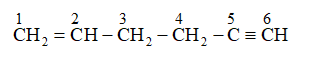In the given organic compound according to IUPAC nomenclature numbering start with double bond side. the carbon atoms numbered as $1,2,3,4,5$, and 6 are $s p^{2}, s p^{2}, s p^{3}, s p^{3}, s p$, and sp hybridized respectively. Thus, the pair of hybridized orbitals involved in the formation of $\mathrm{C}_{2}-\mathrm{C}_{3}$ bond is $\mathrm{sp}^{2}-\mathrm{sp}^{3}$.

Question 37. In the Lassaigne's test for nitrogen in an organic compound, the Prussian blue colour is obtained due to the formation of:

(A) $\mathrm{Na}_{4}\left[\mathrm{Fe}(\mathrm{CN})_{6}\right]$

(B) $\mathrm{Fe}_{4}\left[\mathrm{Fe}(\mathrm{CN})_{6}\right]_{3}$

(C) $\mathrm{Fe}_{2}\left[\mathrm{Fe}(\mathrm{CN})_{6}\right]$

(D) $\mathrm{Fe}_{3}\left[\mathrm{Fe}(\mathrm{CN})_{6}\right]_{4}$

Solution: In the Lassaigne's test for nitrogen in an organic compound, the sodium fusion extract is boiled with iron (II) sulphate and then acidified with sulphuric acid. In the process, sodium cyanide first reacts with iron (II) sulphate and forms sodium hexacyanoferrate (II). Then, on heating with

sulphuric acid, some iron (II) gets oxidised to form iron (III) hexacyanoferrate (II), which is Prussian blue in colour. The chemical equations involved in the reaction can be represented as

$6 \mathrm{CN}^{-}+\mathrm{Fe}^{2+} \rightarrow\left[\mathrm{Fe}(\mathrm{CN})_{6}\right]^{4-}$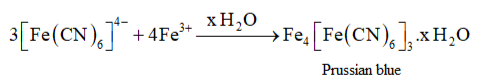Hence, the Prussian blue colour is due to the formation of $\mathrm{Fe}_{4}\left[\mathrm{Fe}(\mathrm{CN})_{6}\right]_{3}$

Question 38. Which of the following carbocation is most stable?

(A) $\left(\mathrm{CH}_{3}\right)_{3} \mathrm{C} . \stackrel{+}{\mathrm{C}} \mathrm{H}_{2}$

(B) $\left(\mathrm{CH}_{3}\right)_{3} \stackrel{+}{\mathrm{C}}$

(C) $\mathrm{CH}_{3} \mathrm{CH}_{2}^{+} \mathrm{CH}_{2}$

(D) $\mathrm{CH}_{3}{ }_{3}^{+} \mathrm{CH} \mathrm{CH}_{2} \mathrm{CH}_{3}$

Solution: $\left(\mathrm{CH}_{3}\right)_{3} \mathrm${ }_{3}^{+} \mathrm$is a tertiary carbocation. A tertiary carbocation is the most stable carbocation due to the electron releasing effect of three methyl groups. An increased$+\mathrm{I}$effect by three methyl groups stabilizes the positive charge on the carbocation. Question 39. The best and latest technique for isolation, purification and separation of organic compounds is: (A) Crystallisation (B) Distillation (C) Sublimation (D) Chromatography Solution: Chromatography is the most useful and the latest technique of separation and purification of organic compounds. It was first used to separate a mixture of coloured substances. Question 40. The reaction:$\mathrm{CH}_{3} \mathrm{CH}_{2} \mathrm{I}+\mathrm{KOH}(\mathrm{aq}) \rightarrow \mathrm{CH}_{3} \mathrm{CH}_{2} \mathrm{OH}+\mathrm{KI}$is classified as: (A) electrophilic substitution (B) nucleophilic substitution (C) elimination (D) addition Solutiom:$\mathrm{CH}_{3} \mathrm{CH}_{2}(\mathrm{l})+\mathrm{KOH}((\mathrm{aq})) \rightarrow \mathrm{CH}_{3} \mathrm{CH}_{2} \mathrm{OH}+\mathrm{Kl}$It is an example of nucleophilic substitution reaction. The hydroxyl group of$\mathrm{KOH}\left(\mathrm{OH}^{-}\right)$with a lone pair of itself acts as a nucleophile and substitutes iodide ion in$\mathrm{CH}_{3} \mathrm{CH}_{2} \mathrm{I}$to form ethanol.${ }_{3}^{+} \mathrm